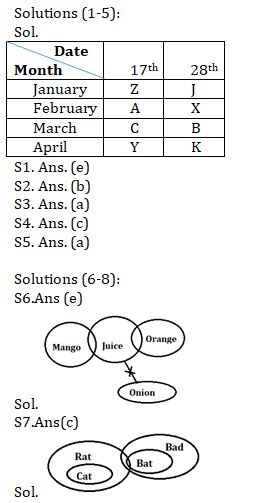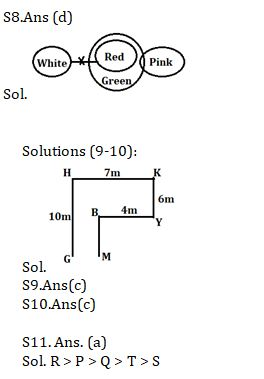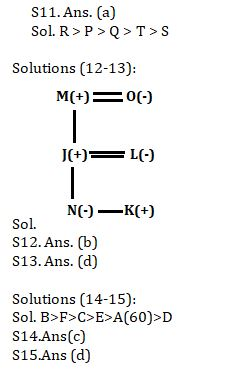Latest Banking jobs   »   Reasoning Ability Quiz For IBPS RRB...

# Reasoning Ability Quiz For IBPS RRB PO, Clerk Prelims 2021- 13th August

Directions (1-5): Study the information carefully and answer the questions given below.
Eight persons X, Y, Z, A, B, C, J and K attend lectures on two different dates 17th and 28th of four different months viz. January, February, March and April of the same year.
J attend lecture on an even date before X. Two persons attend lecture between X and Y. Z attends lecture before C. K attends lecture after Y. X attends lecture in the month which has least number of days. Three persons attend lecture between Z and C. Z does not attends lecture on an even number date. B does not attend lecture in the month having least number of days. Y does not attend lecture before B.

Q1. How many persons attends lecture between X and C?
(a) One
(b) Two
(c) Three
(d) Four
(e) No one

Q2. Who among the following attends lecture on 28th March?
(a) Y
(b) B
(c) K
(d) X
(e) Z

Q3. Who among the following attends lecture immediately after X?
(a) C
(b) A
(c) B
(d) Z
(e)None of these

Q4. Four among the following five belongs to a group. Who does not belong to the group?
(a) J
(b) X
(c) A
(d) B
(e) K

Q5. Who among the following attends lecture on 17th of April?
(a) Y
(b) K
(c) Z
(d) A
(e) B

Directions (6-8): In each of the questions below are given some statements followed by some Conclusions. You have to take the given statements to be true even, if they seem to be at variance from commonly known facts. Read all the conclusions and then decide which of the given conclusions logically follows from the given statements disregarding commonly known facts.
(a) If both conclusions I and II follow.
(b) If either conclusion I or II follows.
(c) If neither conclusion I nor II follows.
(d) If only conclusion II follows.
(e) If only conclusion I follows.

Q6. Statements:
Only a few Mango is Juice
Some juice are Orange
No Juice is Onion
Conclusion:
I: All orange can never be onion
II: All mango can be onion

Q7. Statements:
Only rat is cat
Some rat is bat
Conclusion:
I: Some cat is bad is a possibility
II: All Rat is Bat is a possibility

Q8. Statements:
All Red are Green
Some green are Pink
No Red is White
Conclusions:
I. Some Pink are not white
II. Some Green are not white

Directions (9-10): Study the information carefully and answer the questions given below.
A person starts walking from point G he walks 10m north and reach at point H. From point H, He walks 7m in east direction and reach at point K. From point K, he walks 6m south and reach at point Y. Now he takes a right turn and walks 4m to reach at point B. After reaching at point B he start walking in south direction and reach at point M, which is east of point G.

Q9. What is the shortest distance between point B and point M?
(a) 6m
(b) 7m
(c) 4m
(d) 3m
(e) None of these

Q10. If point S is 5m east of point M than in which direction point S with respect to point K?
(a) North
(b) South
(c) South east
(d) South west
(e) None of these

Q11. There are five persons i.e. P, Q, R, S and T. If S is shorter than T and P. Q is taller than T who is not the taller than P. Q is taller than only two persons. P is not the tallest, then who is the second tallest person among all?
(a) P
(b)S
(c) Q
(d) R
(e)None of these

Directions (12-13): Study the information carefully and answer the questions given below.
J, K, L, M, N and O are six members of a family. There are equal numbers of male and female in the family. J is the son of M and N is the daughter of J. K is the child of L who is the mother of N. O is paternal grandmother of K.

Q12.How is O related to L?
(a)Daughter-in-law
(b)Mother-in-law
(c)Mother
(d)Father-in-law
(e)Father

Q13.How is K related to M?
(a)Daughter
(b)Father
(c)Son
(d)Grandson
(e)Granddaughter

Directions (14-15): Study the following information carefully and answer the given questions.
Amongst Six friends A, B, C, D, E and F, each got different marks in the examination. E score more than A. C score less than F. More than three person score less than B. E does not score more than C. A does not score lowest marks and F does not score highest marks. The one who scores second lowest score 60 marks.

Q14. Who among the following person score fourth highest?
(a) C
(b) A
(c) E
(d) D
(e) None of these

Q15. If C score 80 marks, then what may be the score of E?
(a) 63 marks
(b) 70 marks
(c) 52 marks
(d) Either (a) or (b)
(e) Can’t be determined

Solutions:#### Congratulations!Union Budget 2023-24: Free PDF